# Solve the initial boundary value problem:= a?uzu(0,t) 0r(1, t)hu(1, t) = 1u(x, 0) = sin(Ta

Question
8 views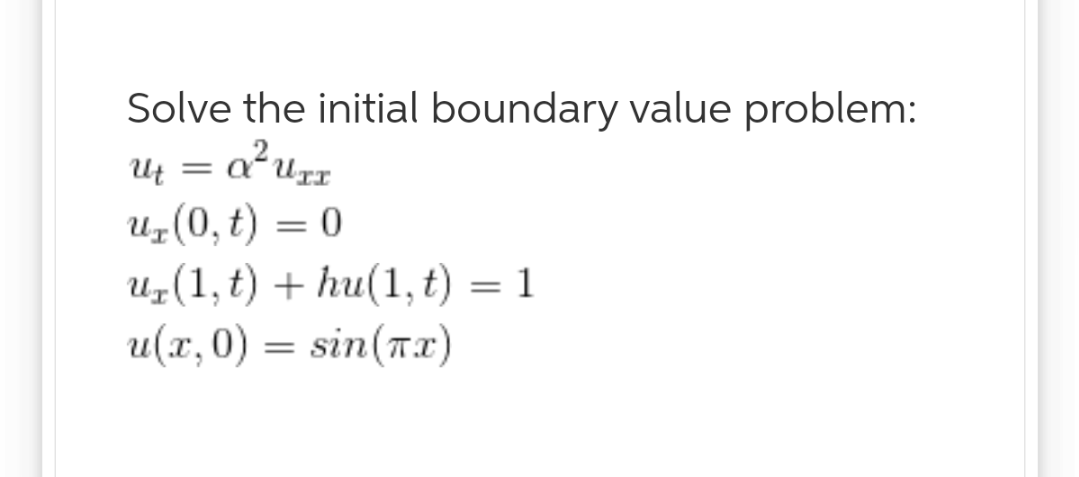help_outlineImage TranscriptioncloseSolve the initial boundary value problem: = a?uz u(0,t) 0 r(1, t)hu(1, t) = 1 u(x, 0) = sin(Ta fullscreen
check_circle

Step 1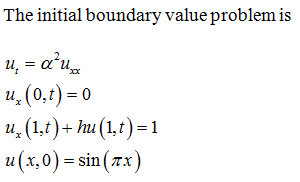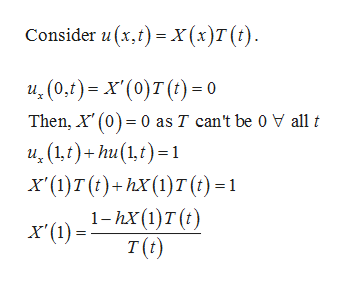help_outlineImage TranscriptioncloseConsider u (x,t) x(x)T (t) u(0,f)= x'(0)T ()0 Then, X'(0) 0 as T can't be 0 V all t и, (14)+ һu(1€) -1 x ()т()-һх()т() -1 1-пx (1)т() T (t) fullscreen
Step 2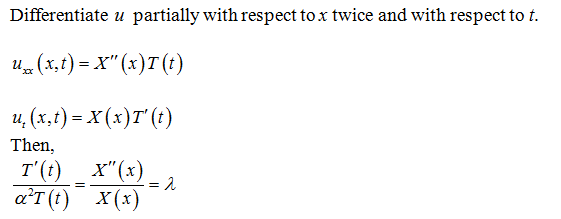...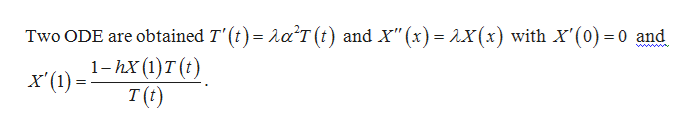help_outlineImage TranscriptioncloseTwo ODE are obtained T'(a T (t) and X" (x) x(x) with X'(0) 0 and = = గ 1-hx (1)T (t) x'(1) T (t) fullscreen

### Want to see the full answer?

See Solution

#### Want to see this answer and more?

Solutions are written by subject experts who are available 24/7. Questions are typically answered within 1 hour.*

See Solution
*Response times may vary by subject and question.
Tagged in

### Math